# LOG#166. D-dimensional laws(I).

My next thread of nested articles are aimed to explore (shallowly, only) the issue of extra dimensions (of space and time!).

Let me begin with the Gauss law for the electric field. The electrical flux in D-dimensional space reads, for radial symmetry,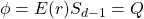up to a multiplicative constant, related toin D=4 spacetime. There,is the radial electric field,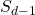is certain gaussian hypersurface containing the electric charge Q. If we take a hypersphere, soequals the surface of the (d-1)-sphere, we would obtain a Coulomb-like law in D=d-1 dimensions:

(1)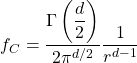Note that we have used units in which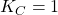for simplicity. We recover the 3D-space, 4D-spacetime result using the above expression with care. You can also check that the electric field can be derived from the potential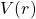through the usual definition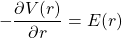How can we modify this law if we include the possibility of having massive gauge bosons and their interactions? If there is a massive gauge boson exchange, then the short-range Yukawa interaction provides the following potential in (d-1)-dimensional space:

(2)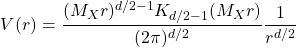and where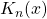is the Bessel function of 2nd type.

Let me remember you some facts about hyperspheres. The (d-1)D hypersphere surface can be computed as follows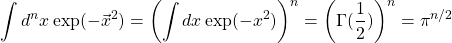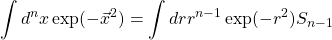and thus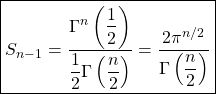The volume for the hypersphere is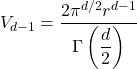where the (d-1)-spherical shell volume is constrained by the relationshipand the solid sphere is instead the constrainIn general (d+1)=D spacetime, for a single point particle, we write the D-dimensional Coulomb-like electric field as

(3)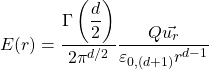By dimensional analysis, we get that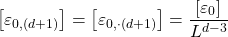Moreover, you have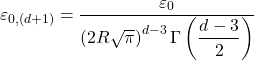to be more precise for any.

Thus, the Coulomb law between two point particles in extra dimensions (XD), D=(d+1)D spacetime reads

(4)and thus the electrostatic (d+1)D field and scalar potential are given by

(5)(6)Magnetism can be also generalized to higher dimensions. A more appropiate language would be the language of differential forms (exterior calculus) and/or geometric calculus (geometric algebra) in order to include not only point particles, but also defects such as branes and their electric/magnetic charges, the notion of duality and more (such as topological terms like Chern-Simons and their nontrivial couplings). But I will write about it in the future, I promise, …Today we will focus on a very basic high-school/lower undergraduate calculus…Forgive me this today. I think it has its advantages for pedagogical reasons to begin at basic level…

The (d+1)D magnetic field law can be written as follows

(7)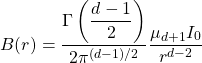A dimensional check provides that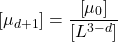and the above law can be understood as the (d+1)D magnetic field inducing a magnetic force. You can also check thatFor one steady current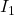over other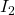parallel (both filamentary) the magnetic force per unit length equals to

(8)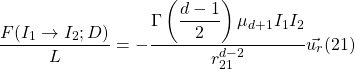Some simple tensor magnetic field equations in (d+1)D, with current distribute over a one dimensional, a two dimensional or a three dimensional cross section, can be guessed. For a 1-dimensional cross section

(9)For a two dimensional cross section current

(10)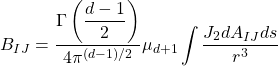and for the three dimensional cross section current

(11)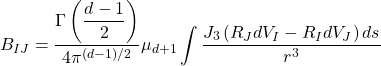and where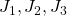are current densities, andis along the current.

Remark: In the 3+1 world we recover the classical and common result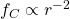andas you can easily check out. For a XD (eXtra Dimensional) world you have, in general, certain lagrangian living on D=d+1 spacetime. A (d+1) Poisson equation readsFree space solutions of the Laplace equation above requires a Fourier transform, so we calculate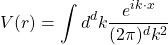and it implies an integral representation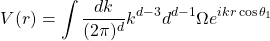and whereis the (d-1)D angular element ((d-1)-dimensional solid angle). We can define spherical coordinatesso we have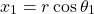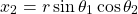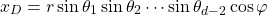and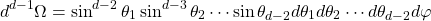Performing the integration carefully, taking into account the surface of the hypersphere, we have an integraland from this, we recover the classical (d+1)-dimensional potential given above, i.e.,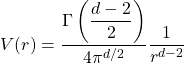Q.E.D.

This site uses Akismet to reduce spam. Learn how your comment data is processed.